Next: The theorem Up: Statistical mechanics Previous: Specification of the state

## The principle of equal a priori probabilities

We now know how to specify the instantaneous state of a many particle system. In principle, such a system is completely deterministic. Once we know the initial state and the equations of motion (or the Hamiltonian) we can evolve the system forward in time and, thereby, determine all future states. In reality, it is quite impossible to specify the initial state or the equations of motion to sufficient accuracy for this method to have any chance of working. Furthermore, even if it were possible, it would still not be a practical proposition to evolve the equations of motion. Remember that we are typically dealing with systems containing Avogadro's number of particles: i.e., about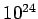particles. We cannot evolvesimultaneous differential equations! Even if we could, we would not want to. After all, we are not particularly interested in the motions of individual particles. What we really want is statistical information regarding the motions of all particles in the system.

Clearly, what is required here is a statistical treatment of the problem. Instead of focusing on a single system, let us proceed in the usual manner and consider a statistical ensemble consisting of a large number of identical systems. In general, these systems are distributed over many different states at any given time. In order to evaluate the probability that the system possesses a particular property, we merely need to find the number of systems in the ensemble which exhibit this property, and then divide by the total number of systems, in the limit as the latter number tends to infinity.

We can usually place some general constraints on the system. Typically, we know the total energy, the total volume, and the total number of particles. To be more honest, we can only really say that the total energy lies betweenand, etc., where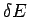is an experimental error. Thus, we only need concern ourselves with those systems in the ensemble exhibiting states which are consistent with the known constraints. We call these the states accessible to the system. In general, there are a great many such states.

We now need to calculate the probability of the system being found in each of its accessible states. Well, perhaps calculate'' is the wrong word. The only way we could calculate these probabilities would be to evolve all of the systems in the ensemble and observe how long on average they spend in each accessible state. But, as we have already mentioned, such a calculation is completely out of the question. So what do we do instead? Well, we effectively guess the probabilities.

Let us consider an isolated system in equilibrium. In this situation, we would expect the probability of the system being found in one of its accessible states to be independent of time. This implies that the statistical ensemble does not evolve with time. Individual systems in the ensemble will constantly change state, but the average number of systems in any given state should remain constant. Thus, all macroscopic parameters describing the system, such as the energy and the volume, should also remain constant. There is nothing in the laws of mechanics which would lead us to suppose that the system will be found more often in one of its accessible states than in another. We assume, therefore, that the system is equally likely to be found in any of its accessible states. This is called the assumption of equal a priori probabilities, and lies at the very heart of statistical mechanics.

In fact, we use assumptions like this all of the time without really thinking about them. Suppose that we were asked to pick a card at random from a well-shuffled pack. I think that most people would accept that we have an equal probability of picking any card in the pack. There is nothing which would favour one particular card over all of the others. So, since there are fifty-two cards in a normal pack, we would expect the probability of picking the Ace of Spades, say, to be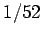. We could now place some constraints on the system. For instance, we could only count red cards, in which case the probability of picking the Ace of Hearts, say, would be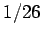, by the same reasoning. In both cases, we have used the principle of equal a priori probabilities. People really believe that this principle applies to games of chance such as cards, dice, and roulette. In fact, if the principle were found not to apply to a particular game most people would assume that the game was crooked.'' But, imagine trying to prove that the principle actually does apply to a game of cards. This would be very difficult! We would have to show that the way most people shuffle cards is effective at randomizing their order. A convincing study would have to be part mathematics and part psychology!

In statistical mechanics, we treat a many particle system a bit like an extremely large game of cards. Each accessible state corresponds to one of the cards in the pack. The interactions between particles cause the system to continually change state. This is equivalent to constantly shuffling the pack. Finally, an observation of the state of the system is like picking a card at random from the pack. The principle of equal a priori probabilities then boils down to saying that we have an equal chance of choosing any particular card.

It is, unfortunately, impossible to prove with mathematical rigor that the principle of equal a priori probabilities applies to many-particle systems. Over the years, many people have attempted this proof, and all have failed miserably. Not surprisingly, therefore, statistical mechanics was greeted with a great deal of scepticism when it was first proposed just over one hundred years ago. One of the its main proponents, Ludvig Boltzmann, got so fed up with all of the criticism that he eventually threw himself off a bridge! Nowadays, statistical mechanics is completely accepted into the cannon of physics. The reason for this is quite simple: it works!

It is actually possible to formulate a reasonably convincing scientific case for the principle of equal a priori probabilities. To achieve this we have to make use of the so-called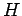theorem.Next: The theorem Up: Statistical mechanics Previous: Specification of the state
Richard Fitzpatrick 2006-02-02